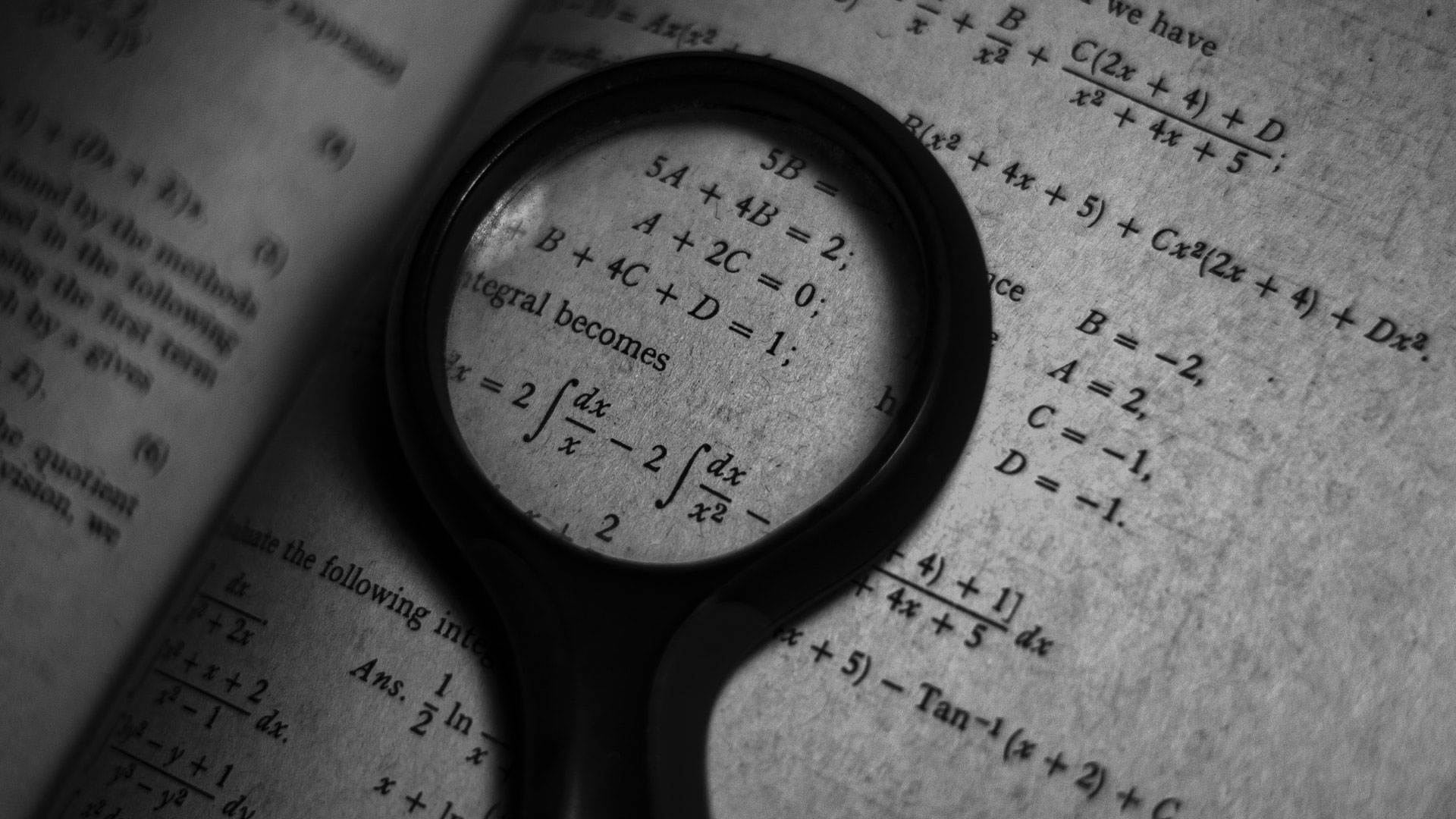Fact checked# What are the Different Disciplines of Mathematics?

Algebra, geometry, trigonometry, calculus, statistics, and probability are all common mathematics disciplines. Most people start...
Mathematics4 minutesMathematics includes many disciplines that have evolved throughout history. Simple examples include addition and subtraction, while extremely complex forms include chaos and games theories. Traditionally, however, the high school and early college years focus on the following disciplines.

Algebra is the gateway to most mathematics studies. Students may study algebra in 8th, 9th, or 10th grade, depending on their skills and school requirements. Normally, first year algebra concerns itself with the study of equations to find unknown elements. Students learn how to solve equations for two to three variables, depending on the class.

Generally, the study of algebra is followed by the study of geometry, which is usually taken after a first year algebra course. A second year algebra course includes geometric principals. In colleges, the study of algebra is often combined with the study of geometry, and students do not take a separate course.

Most teachers start teaching basic algebraic and geometric concepts long before students actually take those classes. In many cases, students are now doing one and two-step equations solving for a variable by third or fourth grade. The familiarity with solving equations is thought to help prepare the student for working with multiple variables in first year algebra.

Students also often learn basic formulas for measuring objects, like triangles, squares, and circles, by fifth or sixth grade. This early preparation in mathematics rarely questions the “why” of a formula, but prepares the students to ask it. This question will be answered by the proofs and theorems that dictate and explain why formulas for obtaining measurements in shapes actually work.

Some students end their mathematics education with second year algebra. Many, however, go on to study trigonometry, a branch that deals with the principles of angles and shapes. Some feel that trigonometry is advanced geometry, while others argue it is a completely separate area of study. It has broad applications, but one most familiar to people is its use in astronomy to measure the distance of stars and planets from each other in a process called triangulation.

After trigonometry, students often study calculus, which is developed from advanced algebra and geometry. In many colleges, students can study either calculus or trigonometry as a final mathematics course. Calculus is actually two different branches: differential and integral. Differential calculus concerns itself with equations measuring things like distance and velocity. Integral calculus evaluates geometry with attention to real world applications, like how time or temperature might affect an equation.

Both forms of calculus are essential to understanding applications in major sciences like physics. In fact, in most colleges, one must at least be eligible to take calculus courses prior to enrolling in physics courses. Some advanced science courses require that one has already taken calculus, since calculus equations are fundamental to understanding the more complex aspects of a science.

Another branch of mathematics is the field of statistics and probability. Those studying economics or accounting must usually take a course in one or the other — or in both — to meet graduation requirements for college. Beyond these branches, there are many other sub-fields that become very specific in their applications. Math majors will pursue these courses in order to earn advanced degrees.

Do you need one-on-one math tutoring? Get matched with an online math tutor by speaking with our academic advisor today!### Get started with a custom-matched tutor for your child.